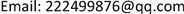HJCEHans Journal of Civil Engineering2326-3458Scientific Research Publishing10.12677/HJCE.2019.82050HJCE-29453HJCE20190200000_95127056.pdf工程技术 基于ANSYS蜂巢约束路基边坡稳定性分析 Stability Analysis of Honeycomb Confined Subgrade Slope Based on ANSYS 21维刚3141海权15显威21川和21中建丝路建设投资有限公司，陕西 西安哈尔滨工业大学交通科学与工程学院，黑龙江 哈尔滨黑龙江省八达路桥建设有限公司，黑龙江 哈尔滨null中交公路规划设计院有限公司，北京030220190802430441© Copyright 2014 by authors and Scientific Research Publishing Inc. 2014This work is licensed under the Creative Commons Attribution International License (CC BY). http://creativecommons.org/licenses/by/4.0/

ANSYS，蜂巢约束，路基边坡，坡度，蜂巢高度, ANSYS Honeycomb Constraints Subgrade Slope Slope Honeycomb Height

1哈尔滨工业大学交通科学与工程学院，黑龙江 哈尔滨

2黑龙江省八达路桥建设有限公司，黑龙江 哈尔滨

3中建丝路建设投资有限公司，陕西 西安

4中交公路规划设计院有限公司，北京Copyright © 2019 by author(s) and Hans Publishers Inc.

This work is licensed under the Creative Commons Attribution International License (CC BY).

http://creativecommons.org/licenses/by/4.0/1. 引言

2. Drucker-Prager弹塑性模型建立

3. 蜂巢约束路基对边坡稳定性影响分析3.1. 无蜂巢约束系统时的边坡稳定性分析

The model siz

GK-1H1 = 2 m, H2 = 2 mGK-6H1 = 2 m, H2 = 2 m
L1 = 10 m, L2 = 15.15 mL1 = 10 m, L2 = 14.15 m
GK-2H1 = 4 m, H2 = 2 mGK-7H1 = 4 m, H2 = 2 m
L1 = 10 m, L2 = 18.15 mL1 = 10 m, L2 = 16.15 m
GK-3H1 = 6 m, H2 = 2 mGK-8H1 = 6 m, H2 = 2 m
L1 = 10 m, L2 = 21.15 mL1 = 10 m, L2 = 18.15 m
GK-4H1 = 8 m, H2 = 2 mGK-9H1 = 8 m, H2 = 2 m
L1 = 10 m, L2 = 24.15 mL1 = 10 m, L2 = 20.15 m
GK-5H1 = 10 m, H2 = 2 mGK-10H1 = 10 m, H2 = 2 m
L1 = 10 m, L2 = 27.15 mL1 = 10 m, L2 = 22.15 m

Material parameter

1800400.353028

1507500.3

800142100.34

3.2. 有蜂巢约束系统时边坡稳定性分析

3.3. 蜂巢约束系统对边坡稳定性的影响分析

4. 降雨对蜂巢约束路基边坡稳定性的影响分析

Material parameters of subgrade soil soaked in wate

2300400.352019

Slope stability coefficient of soil-soaked subgrad
i = 1.5i = 1.0

5. 蜂巢结构尺寸对边坡稳定性的影响分析

6. 结论

1) 对比无约束的边坡，边坡附加蜂巢约束系统后，边坡失稳滑动面由坡脚处移动到坡脚前，并且滑动面均通过路基顶面，没有出现三角形的滑移区。同时，边坡坡度i = 1.5时，边坡的稳定性系数分别增加了4.7%~5.9%；在边坡坡度i = 1.0时，边坡的稳定性系数分别增15.8%~20%，边坡的稳定性有明显提高。

2) 其次，研究发现，蜂巢系统可以减小路基失稳滑动面区域内的路基顶面变形，而对滑动区域以外的路基顶面的竖向变形没有影响，尤其对边坡较陡的情况更为明显。在边坡坡度i = 1.0时，附加了蜂巢系统之后最大可以减小14.8%的坡顶竖向变形。

3) 最后，在研究蜂巢高度对边坡稳定性影响时发现，在蜂巢高度为5 cm时，蜂巢对边坡的稳定性没有加强作用，这时与没有蜂巢系统时的边坡稳定性系数相同，随着蜂巢高度的增加，边坡的稳定性系数呈现线性的增加趋势，因此在考虑边坡稳定的情况下，在参照蜂巢系统材料成本的前提下，应尽量选用较高的蜂巢约束系统。